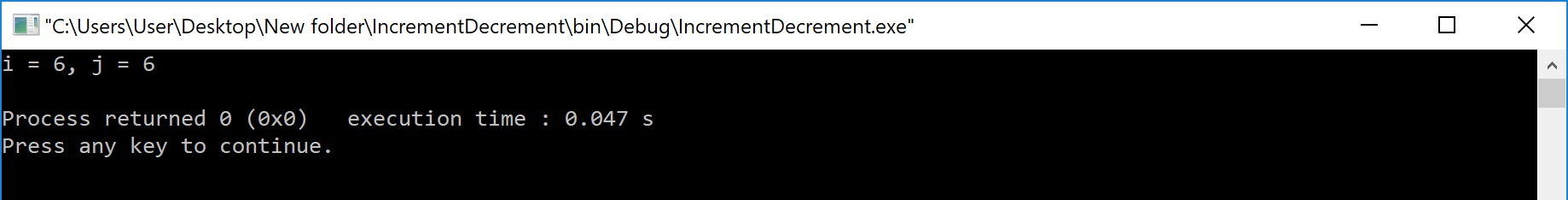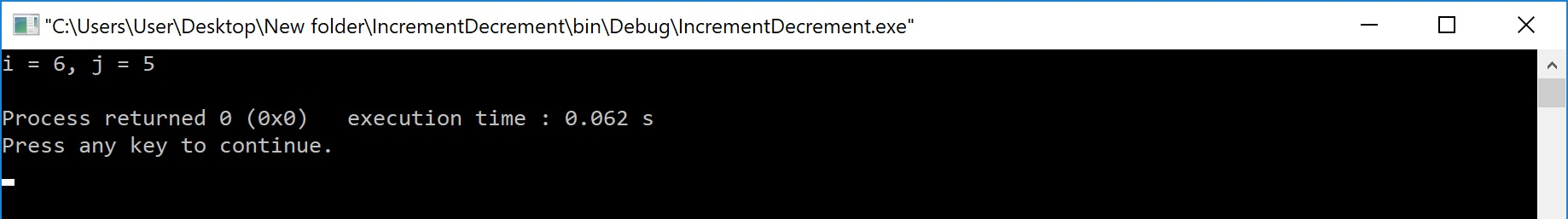The perfect place for easy learning...

×

# C Operators

An operator is a symbol used to perform arithmetic and logical operations in a program. That means an operator is a special symbol that tells the compiler to perform mathematical or logical operations. C programming language supports a rich set of operators that are classified as follows.

1. Arithmetic Operators
2. Relational Operators
3. Logical Operators
4. Increment & Decrement Operators
5. Assignment Operators
6. Bitwise Operators
7. Conditional Operator
8. Special Operators

# Arithmetic Operators (+, -, *, /, %)

The arithmetic operators are the symbols that are used to perform basic mathematical operations like addition, subtraction, multiplication, division and percentage modulo. The following table provides information about arithmetic operators.

Operator Meaning Example
+ Addition 10 + 5 = 15
- Subtraction 10 - 5 = 5
* Multiplication 10 * 5 = 50
/ Division 10 / 5 = 2
% Remainder of the Division 5 % 2 = 1

The addition operator can be used with numerical data types and character data type. When it is used with numerical values, it performs mathematical addition and when it is used with character data type values, it performs concatination (appending).

The remainder of the division operator is used with integer data type only.

# Relational Operators (<, >, <=, >=, ==, !=)

The relational operators are the symbols that are used to compare two values. That means the relational operators are used to check the relationship between two values. Every relational operator has two results TRUE or FALSE. In simple words, the relational operators are used to define conditions in a program. The following table provides information about relational operators.

Operator Meaning Example
< Returns TRUE if the first value is smaller than second value otherwise returns FALSE 10 < 5 is FALSE
> Returns TRUE if the first value is larger than second value otherwise returns FALSE 10 > 5 is TRUE
<= Returns TRUE if the first value is smaller than or equal to second value otherwise returns FALSE 10 <= 5 is FALSE
>= Returns TRUE if the first value is larger than or equal to second value otherwise returns FALSE 10 >= 5 is TRUE
== Returns TRUE if both values are equal otherwise returns FALSE 10 == 5 is FALSE
!= Returns TRUE if both values are not equal otherwise returns FALSE 10 != 5 is TRUE

# Logical Operators (&&, ||, !)

The logical operators are the symbols that are used to combine multiple conditions into one condition. The following table provides information about logical operators.

Operator Meaning Example
&& Logical AND - Returns TRUE if all conditions are TRUE otherwise returns FALSE 10 < 5 && 12 > 10 is FALSE
|| Logical OR - Returns FALSE if all conditions are FALSE otherwise returns TRUE 10 < 5 || 12 > 10 is TRUE
! Logical NOT - Returns TRUE if condition is FLASE and returns FALSE if it is TRUE !(10 < 5 && 12 > 10) is TRUE

⇒ Logical AND - Returns TRUE only if all conditions are TRUE, if any of the conditions is FALSE then complete condition becomes FALSE.
⇒ Logical OR - Returns FALSE only if all conditions are FALSE, if any of the conditions is TRUE then complete condition becomes TRUE.

# Increment & Decrement Operators (++ & --)

The increment and decrement operators are called unary operators because both need only one operand. The increment operators adds one to the existing value of the operand and the decrement operator subtracts one from the existing value of the operand. The following table provides information about increment and decrement operators.

Operator Meaning Example
++ Increment - Adds one to existing value int a = 5;
a++; ⇒ a = 6
-- Decrement - Subtracts one from existing value int a = 5;
a--; ⇒ a = 4

The increment and decrement operators are used infront of the operand (++a) or after the operand (a++). If it is used infront of the operand, we call it as pre-increment or pre-decrement and if it is used after the operand, we call it as post-increment or post-decrement.

# Pre-Increment or Pre-Decrement

In the case of pre-increment, the value of the variable is increased by one before the expression evaluation. In the case of pre-decrement, the value of the variable is decreased by one before the expression evaluation. That means, when we use pre-increment or pre-decrement, first the value of the variable is incremented or decremented by one, then the modified value is used in the expression evaluation.

# Example Program

``````#include<stdio.h>
#include<conio.h>

void main(){
int i = 5,j;

j = ++i; // Pre-Increment

printf("i = %d, j = %d",i,j);

}```
```

Output:# Post-Increment or Post-Decrement

In the case of post-increment, the value of the variable is increased by one after the expression evaluation. In the case of post-decrement, the value of the variable is decreased by one after the expression evaluation. That means, when we use post-increment or post-decrement, first the expression is evaluated with existing value, then the value of the variable is incremented or decremented by one.

# Example Program

``````#include<stdio.h>
#include<conio.h>

void main(){
int i = 5,j;

j = i++;  // Post-Increment

printf("i = %d, j = %d",i,j);

}```
```

Output:# Assignment Operators (=, +=, -=, *=, /=, %=)

The assignment operators are used to assign right-hand side value (Rvalue) to the left-hand side variable (Lvalue). The assignment operator is used in different variants along with arithmetic operators. The following table describes all the assignment operators in the C programming language.

Operator Meaning Example
= Assign the right-hand side value to left-hand side variable     A = 15
+= Add both left and right-hand side values and store the result into left-hand side variable     A += 10
⇒ A = A+10
-= Subtract right-hand side value from left-hand side variable value and store the result
into left-hand side variable
A -= B
⇒ A = A-B
*= Multiply right-hand side value with left-hand side variable value and store the result
into left-hand side variable
A *= B
⇒ A = A*B
/= Divide left-hand side variable value with right-hand side variable value and store the result
into the left-hand side variable
A /= B
⇒ A = A/B
%= Divide left-hand side variable value with right-hand side variable value and store the remainder
into the left-hand side variable
A %= B
⇒ A = A%B

# Bitwise Operators (&, |, ^, ~, >>, <<)

The bitwise operators are used to perform bit-level operations in the c programming language. When we use the bitwise operators, the operations are performed based on the binary values. The following table describes all the bitwise operators in the C programming language.
Let us consider two variables A and B as A = 25 (11001) and B = 20 (10100).

Operator Meaning Example
& the result of Bitwise AND is 1 if all the bits are 1 otherwise it is 0     A & B
⇒ 16 (10000)
| the result of Bitwise OR is 0 if all the bits are 0 otherwise it is 1     A | B
⇒ 29 (11101)
^ the result of Bitwise XOR is 0 if all the bits are same otherwise it is 1     A ^ B
⇒ 13 (01101)
~ the result of Bitwise once complement is negation of the bit (Flipping)     ~A
⇒ 6 (00110)
<< the Bitwise left shift operator shifts all the bits to the left by the specified number of positions     A << 2
⇒ 100 (1100100)
>> the Bitwise right shift operator shifts all the bits to the right by the specified number of positions     A >> 2
⇒ 6 (00110)

# Conditional Operator (?:)

The conditional operator is also called a ternary operator because it requires three operands. This operator is used for decision making. In this operator, first we verify a condition, then we perform one operation out of the two operations based on the condition result. If the condition is TRUE the first option is performed, if the condition is FALSE the second option is performed. The conditional operator is used with the following syntax.

# Special Operators (sizeof, pointer, comma, dot, etc.)

The following are the special operators in c programming language.

# sizeof operator

This operator is used to find the size of the memory (in bytes) allocated for a variable. This operator is used with the following syntax.

# Pointer operator (*)

This operator is used to define pointer variables in c programming language.

# Comma operator (,)

This operator is used to separate variables while they are declaring, separate the expressions in function calls, etc.

# Dot operator (.)

This operator is used to access members of structure or union.